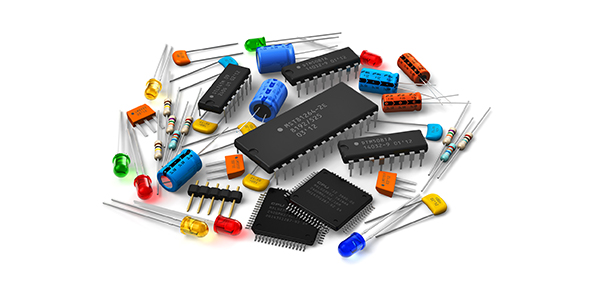# Assemble And Disassemble

50 Questions | Attempts: 721
ShareSettings• 1.
Is the electronic component that removes the ripples of the power supply output?
• A.

Transistor

• B.

Resistor

• C.

Diode

• D.

Capacitor

• 2.
Is the essential components of a circuit are?
• A.

• B.

Inductive reactance

• C.

Hysteresis loss

• D.

Forward bias

• 3.
Is the limited core is used in transformers to minimize ?
• A.

• B.

Polarity

• C.

Hysteresis loss

• D.

Number of effective junctions

• 4.
Is the filter capacitor used in a power supply ?
• A.

Forward bias

• B.

Electronic type

• C.

Series where current is to be determined

• D.

Transistor

• 5.
Is the opposition to the flow of a.c offered  by the coil is called ?
• A.

Forward bias

• B.

Inductive reactance

• C.

Emitter and collector

• D.

Polarity

• 6.
Is the diode, that anode is connected to the positive side of the source while the cathode is
• A.

Forward bias

• B.

Meter sensitivity

• C.

Rosin core soldier

• D.

Polarity

• 7.
Is the most important consideration when connecting an electrolytic capacitor in a circuit ?
• A.

Series where the current is to be determined

• B.

Emitter and collector

• C.

Polarity

• D.

Meter sensitivity

• 8.
Is the devices the most important thing to know about the vom?
• A.

Meter sensitivity

• B.

Tester

• C.

Polarity

• D.

Number of effective junctions

• 9.
Is the highest resistance in a transistor when cheeked or tested with a vom is between
• A.

Base and collector

• B.

Base and emitter

• C.

Emitter and collector

• D.

Shorted or open transistor

• 10.
Is not a basic component of a typical low voltage power supply?
• A.

Series where the current is to determined

• B.

Collector to emitter

• C.

Base to emitter

• D.

Transistor

• 11.
When measuring current in a circuit the vom connected ?
• A.

Polarity

• B.

Series where the current is to determined

• C.

Number of effective junction

• D.

V=h deflection yoke

• 12.
Is the most commonly used protective device ?
• A.

Resistor

• B.

Fuse

• C.

Capacitor

• D.

Transistor

• 13.
In a code 2SA 180 A the number 2 stands
• A.

Infinite resistance

• B.

Shunt bypass capacitor

• C.

Series where the current is to be determined

• D.

Number of effective junctions

• 14.
Is the AC 187 is a code use?
• A.

European standard

• B.

Infinite resistance

• C.

Cathode burn

• D.

Convergence yoke

• 15.
Is the kind of electronic component is used in treble out circuit to reduced the output at high frequencies ?
• A.

Cathode burn

• B.

Infinite resistance

• C.

Capacitor

• D.

Shunt bypass capacitor

• 16.
To improve the sound of a small capacitor what is the value of capacitor should be connected across the primary winding of the output transformer in treble circuit designs?
• A.

.01 uf

• B.

.001 uf

• C.

.10 uf

• D.

.010 uf

• 17.
In the 0.001 microfarad capacitor is placed in parallel with a 100 pf capacitor the total capacitance ?
• A.

100000 pf

• B.

10000 pf

• C.

1000 pf

• D.

1100 pf

• 18.
Should not be included as a sign of short circuit ?
• A.

Definite resistance

• B.

Infinite reactance

• C.

Infinite resistance

• D.

Definite reactance

• 19.
Is not a sign of short circuit ?
• A.

Definite resistance

• B.

Infinite resistance

• C.

Cathode burn

• D.

V=h deflection yoke

• 20.
Damage to the crt and can not be repaired except replacing crt?
• A.

Convergence yoke

• B.

Resetting the yoke

• C.

Shunt bypass capacitor

• D.

Cathode burn

• 21.
Is the last step  in the final adjustment for color purity?
• A.

Resetting the yoke

• B.

Shunt bypass capacitor

• C.

Infinite resistance

• D.

Cathode burn

• 22.
This magnetic yoke has individual adjustments for each color to make the beams converge?
• A.

Resetting the yoke

• B.

Convergence yoke

• C.

Printed circuit

• D.

V=h deflection yoke

• 23.
That method is applied in order for an anode voltage will not exceed its recommended voltage?
• A.

V=h deflection yoke

• B.

Infinite resistance

• C.

The high voltage is cut off resulting in increase brightness

• D.

Cathode burn

• 24.
The setting the beam landing adjustment correctly electron beams are aimed by moving the ?
• A.

Cathode burn

• B.

Resetting yoke

• C.

V=h deflection yoke

• D.

Rosin-core soldier

• 25.
Is that devices are not advisable electronics assembly and repair work?
• A.

Side cutter pliers

• B.

Rosin core solder

• C.

Cathode burn

• D.

V=h deflection yoke

## Related TopicsBack to top
×

Wait!
Here's an interesting quiz for you.